# Sphere + body volume - examples - page 2

1. GasholderThe gasholder has spherical shape with a diameter 20 m. How many m3 can hold in?
2. Sphere fallHow many percent fall volume of sphere if diameter fall 10×?
3. Spheres in sphere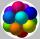How many spheres with a radius of 15 cm can fits into the larger sphere with a radius of 150 cm?
4. Hollow sphere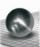The volume of the hollow ball is 3432 cm3. What is its internal radius when the wall thickness is 3 cm?
5. StadiumA domed stadium is in the shape of spherical segment with a base radius of 150 m. The dome must contain a volume of 3500000 m³. Determine the height of the dome at its centre to the nearest tenth of a meter.
6. Inscribed sphereHow many % of the volume of the cube whose edge is 6 meters long is a volume of a sphere inscribed in that cube?
7. Inscribed sphereHow many percents of the cube volume takes the sphere inscribed into it?
8. Equilateral cylinder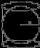A sphere is inserted into the rotating equilateral cylinder (touching the bases and the shell). Prove that the cylinder has both a volume and a surface half larger than an inscribed sphere.
9. Cube, cuboid, and sphereVolumes of a cube and a cuboid are in ratio 3: 2. Volumes of sphere and cuboid are in ratio 1: 3. In what ratio are the volumes of cube, cuboid, and sphere?
10. Cube into sphereThe cube has brushed a sphere as large as possible. Determine how much percent was the waste.
11. Iron ball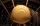The iron ball has a weight of 100 kilograms. Calculate the volume, radius, and surface if the iron's density is h = 7.6g/cm3.
12. InscribedCube is inscribed in the cube. Determine its volume if the edge of the cube is 10 cm long.
13. Hemispherical hollowThe vessel hemispherical hollow is filled with water to a height of 10 cm =. How many liters of water are inside if the inside diameter of the hollow is d = 28cm?
14. Spherical tank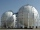The tank of a water tower is a sphere of radius 35ft. If the tank is filled to one quarter of full, what is the height of the water?
15. Sphere floatingWill float a hollow iron ball with an outer diameter d1 = 20cm and an inside diameter d2 = 19cm in the water? The iron density is 7.8 g/cm 3. (Instructions: Calculate the average sphere density and compare with the density of the water. )
16. Sphere VSFind the surface and volume of a sphere that has a radius of 2 dm.
17. Sphere radiusThe radius of the sphere we reduce by 1/3 of the original radius. How much percent does the volume and surface of the sphere change?
18. Volume of ballFind the volume of a volleyball that has a radius of 4 1/2 decimeters. Use 22/7 for π

Do you have an interesting mathematical example that you can't solve it? Enter it, and we can try to solve it.

To this e-mail address, we will reply solution; solved examples are also published here. Please enter e-mail correctly and check whether you don't have a full mailbox.